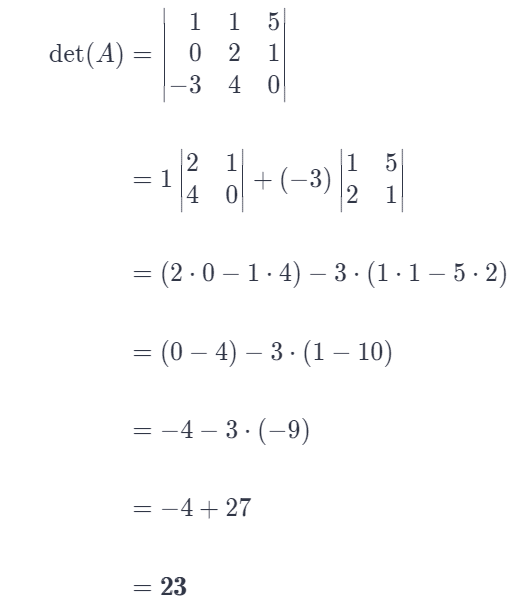# Find The Volume Of The Parallelepiped With One Vertex At The Origin And Adjacent Vertices At

We thoroughly check each answer to a question to provide you with the most correct answers. Found a mistake? Let us know about it through the REPORT button at the bottom of the page.

Find the volume of the parallelepiped with one vertex at the origin and adjacent vertices at (1, 0, -3), (1, 2, 4), and (5, 1, 0).

Answer: Compute the determinant of the matrix whose column entries are coordinates of the adjacent vertices of the given parallelepiped. This determinant represents its volume and is equal to 23.

## Explanation

Finding the volume of the parallelopiped with one vertex in the origin and other adjacent vertices is equivalent to finding the determinant of the matrix whose column entries are coordinates of the corresponding adjacent vertices. Let’s denote the matrix obtained in this way by A. We will find its determinant using cofactor expansion across the first column (because it has one zero-entry, making our job easier).Therefore, the volume of the given parallelopiped is equal to 23.